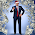Wednesday, April 1, 2015

Coming up: The η 'll gate ya!

$\eta = {A_S \over A_l}$
$A_S=$ Surface Area of the black hole
$A_l=$ Equatorial cross-sectional area of the Planck Spherical Unit volume - a sphere of diameter Planck length $l$.

This number $\eta$ is HUGE!!!  It's related to http://en.wikipedia.org/wiki/Holographic_principle.

Basically, we have to figure out where this $\eta$ came from.  A proper complete derivation would clearly make a logical argument explaining how $\eta={A_S \over A_l}$, however, there is quite a bit of mainstream black hole information theory analysis behind it.

What I am planning on doing is saving for now is save this detailed analysis for later, and proceed with the equations, assuming $\eta={A_S \over A_l}$ is true.

One argument without going into a deep theoretical argument will be made. Since the black hole is floating in the vacuum of space time and the density of the vacuum is WAY higher than that of the black hole, and this vacuum is like a super-fluid ocean that the black hole floats in, then, and considering the fact that matter is simply a resonance in the fabric of space time and matter actally can be said to consist of the fabric of space time itself, however, in a scaled version due to it floating, one might simply see that the units work out, and if the equation matches measurements then we could proceed to see what further developments come out of this approach and what other unsolved physics problems can now be solved and a much deeper insight gained into.

The next area to look at to get more insight will be on the origin of spin, the black hole / white hole space time dynamic. Nassim has worked out this with Elizabeth Rauscher in 2004 and the PDFs can be found here"  http://www.hiup.org/publications

I will be pointing to more specific articles in a futre post concerning the Derivation of MpRp=4LM.

The Surfer, OM-IV

1.2.